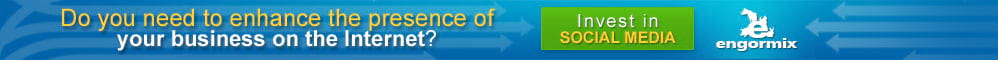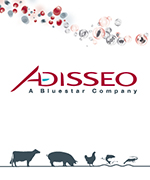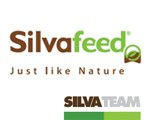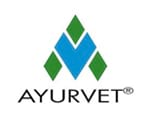# Application of Real Coded Genetic Algorithm (RGA) to Find Least Cost Feedstuffs for Dairy Cattle During Pregnancy

Published on: 11/8/2018
Author/s :
Summary

Background and Objective: The dairy farmers are having limitations in terms of “feedstuffs” available in the tropical region. To meet nutrients requirement at cheapest cost feedstuff need a software programme. There are several techniques/software that have been adopted to meet nutrient requirement of dairy animal. However, there is no particular technique suitable for formulating ration in least cost manner for dairy cattle. Real coded genetic algorithm (RGA) is a better approach than conventional methods as unlike older techniques, it is not easily break even if the inputs are modified and RGA gives significant benefits over large and complex optimization techniques even if it is linear programming problem. Hence, a comparative study was planned with embassies on RGA to find a suitable technique, which is farmer-friendly to be adopted at farm level.

Materials and Methods: The linear programming model (simplex LP, GRG non-linear, Evolutionary Algorithm (EA) and RGA with and without seeding the random number generations) were executed and compared for least cost feed formulation techniques with adopting three different types of cattle's weighing 500 kg each and yielding 10 L milk with 4% of fat content during 7th, 8th and 9th months of pregnancy and considering nutrient requirement on dry matter  basis.

Results: The linear programming model is been solved by simplex LP, GRG non-linear, EA method and RGA with and without seeding the random number generations. There was no significance difference (p>0.05) found with various techniques adopted for feed formulation. However, there will be a better scope for RGA to formulate feed for dairy animals with limited resources at Indian scenario. Conclusion: It could be concluded that real coded genetic algorithm (RGA) can also be used for ration formulation to find least cost feedstuffs in dairy cattle, also we are able to economize the total mixed ration cost such that the feed requirements of the animals are met without any nutrients deficiency.

Key words: Dairy feed, linear programming, least cost, real coded genetic algorithm

INTRODUCTION

As far as the profit is concerned to the farmers, the cost of the feed plays an important role. The dairy farmers in Karnataka are having limitations in terms of feedstuffs available in the region. Farmers faced major challenge to formulate the balanced least cost ration for pregnant cattle at the 3rd trimester where they not only need ration for maintenance of body but also for milk production and fetus growth with the available feedstuffs. There are several techniques software that have been adopted to meet nutrient requirement of dairy animal. However, there is no particular techniques suitable for formulating ration in least cost manner for dairy cattle. Hence, a comparative study is been planned to find a suitable technique, which is farmer friendly to be adopted at farm level. The present study is to formulate the least cost balanced ration for pregnant cattle's at third trimester. The nutrient requirement will differs at 7th, 8th  and 9th months of pregnancy. Nutrients requirement were calculated according to ICAR1, linear programming models did NRC2 at different months of pregnancy with 10 L of milk yield and 4% of fat and least cost feed formulation. The linear programming model is been solved by GRG non-linear, evolutionary alogrithm, simplex LP method and real coded genetic algorithm (RGA) with and without seeding the random number generations3-5.

In 1951, Waugh applied linear programming technique developed by Koopmans to provide the solution of least cost dairy feed6. Therefore, from past 7 decades conventional linear programming method was very popular to solve animal diet problem7-9. Tozer and Stokes10 used multi objective programming to reduce nutrient excretion from dairy cows through incorporation of nutrient excretion function into ration formulation10 and Zhang and Roush11 applied multiple objective programming to the feed formulation of broiler grower ration with the objective of minimizing the nutrient variance and minimizing the ration cost11. Many researchers have introduced a use of computer software and excel solver for solving linear programming problems12-15. While, solving diet problem using LP model mathematically, it only solves primary technical issues, which can have weak relation with economic problem of minimizing or maximizing the difference between costs and feeding over time. To overcome this problem various types of methodologies like: Goal programming, multi-objective programming, non-linear programming etc. is being used since many years16-20. It has showed that linear programming approach to indicate how best available local ingredients can be combined effectively to formulate the least cost ration for broilers21-23. In 2014 combined LP with Goal Programming (GP) for feed formulation displaying the dip in cost as a major advantage of using goal-programming approach24. However, there is no particular techniques is suitable for formulating ration in least cost manner for dairy cattle. Hence, a comparative study is been planned to find suitable technique that is farmer friendly to be adopted at farm level.

MATERIALS AND METHODS

The present study based on primary data collected from Mandya district of Karnataka based on ICAR1 and NRC2 standards23. Briefly, the cows during pregnancy of 3rd trimester need balanced ration for body maintenance, milk production (with minimum of 4% of fat content) and for fetus growth, therefore to formulate the low cost diet for these cows is major challenge for farmers. Therefore, it has been introduced LP models at 3 different months of pregnancy at different body weight. As the growth of fetus increases significantly after 6 months of pregnancy, the nutrient requirement of cattle at 7th, 8th and 9th month of pregnancy is presented in Table 1 and 2. The estimation of nutrient requirements during late pregnancy requires accurate values for rates of nutrient accretion in concepts tissues ICAR1. The ration was formulated using following steps.

Step 1: Calculation of nutrient requirement: The nutrient requirement of cattle1, 2 and 3 is calculated based on body weight, milk yield, fat percentage and pregnancy status. Dry matter intake, crude protein and total digestible nutrients are calculated according to ICAR1, phosphorus and calcium according to NRC2 standards on dry matter basis (Table 1).

Step 2: Selection of ingredients: Based on primary data collected at Mandya district of Karnataka locally available roughages, concentrate and minerals are listed below. Among these ingredients commonly, available dry roughages are paddy straw and ragi straw. Commonly available green roughages are bajra×napier grass (Co-4), multicut sorghum (Co-FS 29) and maize fodder.

Step3: Fixing the constraints: To obtain the least cost feed we have some constraints for each nutrient and it is unique for each animal. The minimum and maximum level of Crude Protein (CP 9.82-11%), Total Digestible Nutrient (TDN 46.73-51%), calcium (0.38-0.8%), phosphorus (0.23-0.5%), roughage (40-80%) and concentrates (20-70%) was calculated on total dry matter intake for each types of cattle. The calculated constraints for each animal are shown below:

Step 4: Finding result: In this step, it was found the least cost of “feedstuffs” after fulfilling constraints assigned by solving linear programming model by various mathematical tools and then translate the obtained result as recommendation to the farmers. Schematic diagram of the methodology followed is shown in Fig. 1.

Mathematical modeling: Linear programming modelofeach cattle consists of one objective function and seven constraints as follows:

LP model for cattle 1:

Minz = 5x1+3x2+3x3+3x4+3x5 +17x6 +38x7 +23x8+23x9+17x10+12x11+21x12+17x13+4x14 +50x15 +28x16+ 5x17

Subjected to:

x1+x2+x3+x4+x5+x6+x7+x8+x9+x10+x11+x12+x13+x14+x15+x16+x17  = 16.75

1.644#0.0513x1+0.08x2+0.08x3+0.07x4+0.06x5+0.081x6+0.42x7+0.22x8+0.32x9+0.12x10+0.17x11+0.16x12+0.22x13#1.8425

7.83#0.4x1+0.52x2+0.6x3+0.5x4+0.42x5+0.792x6+0.7x7+0.7x8+0.7x9+0.7x10+0.6x11+1.1x12+0.7x13#8.5425

0.065#0.0018x1+0.00144x2+0.0053x3+0.0012x4+0.0015x5+0.00018x6+0.00018x7+0.00036x8+0.00036x9+0.01067x10+0.000108x11+0.003x12+0.005x13+0.36x14+0.32x15+0.24x16#0.134

0.04#0.0008x1+0.0009x2+0.0014x3+0.0009x4+0.0009x5+0.0027x6+0.00225x7+0.009x8+0.009x9+0.00093x10+0.00234x11+0.0062x12+0.0045x13+0.06x15+0.16 x16#0.08375

6.7#x1+x2+x3+x4+x5#13.4

3.35#x6+x7+x8+x9+x10+x11+x12+x13+x14+x15+x16+x17#11.725

Bounds on variables:

0.8375#x1#4.1875, 0.8375#x2#4.1875, 0.8375#x3#4.1875, 0.8375#x4#4.1875, 0.8375#x5#4.1875, 0.8375#x6#3.35, 0#x7#4.1875, 0#x8#4.1875, 0#x9#3.35, 0.8375#x100#1.675, 0#x111#3.35, 0#x122#0.8375, 0.8375#x133#3.35, 0#x144#0.1675, 0.067#x155#0.08375, 0#x166#0.0335, 0.134#x177#0.1675

LP model for cattle 2:

Minz = 5x1+3x2+3x3+3x4+3x5 +17x6+38x7 +23x8+23x9+ 17x10+12x11+21x12+17x13+4x14+50x15+28x16+ 5x17

Subjected to:

x1+x2+x3+x4+x5+x6+x7+x8+x9+x10+x11+x12+x13+x14+x15+ x16+x17= 16.89

1.691#0.0513x1+0.08x2+0.08x3+0.07x4+0.06x5+0.081x6+0.42 x7+0.22x8+0.32x9+0.12x10+0.17x11+0.16x12+0.22x13#1.8579

7.93#0.4x1+0.52x2+0.6x3+0.5x4+0.42x5+0.792x6+0.7x7+ 0.7x8+0.7x9+0.7x10+0.6x11+1.1x12+0.7x13#8.6139

0.065#0.0018x1+0.00144x2+0.0053x3+0.0012x4+ 0.0015x5+0.00018x6+0.00018x7+0.00036x8+0.00036x9+0.01067x10+0.000108x11+0.003x12+0.005x13+0.36x14+0.32x15 +0.24x16#0.13512

0.04#0.0008x1+0.0009x2+0.0014x3+0.0009x4+0.0009x5+ 0.0027x6+0.00225x7+0.009x8+0.009x9+0.00093x10+ 0.00234x11+0.0062x12+0.0045x13+0.06x15+0.16x16#0.08445

6.756#x1+x2+x3+x4+x5#13.512

3.378#x6+x7+x8+x9+x10+x11+x12+x13+x14+x15+x16+x17#11.823

Bounds on variables:

0.8445#x1#4.2225, 0.8445#x2#4.2225, 0.8445#x3#4.2225, 0.8445#x4#4.2225, 0.8445#x5#4.2225, 0.8445#x6#3.378, 0#x7#4.2225, 0#x8#4.2225, 0#x9#3.378, 0.8445#x10#1.689, 0#x11#3.378, 0#x12#0.8445, 0.8445#x13#3.378, 0#x14#0.1689, 0.06756#x15#0.8445, 0#x16#0.03378, 0.13512#x17#0.1689

LP model for cattle 3:

Minz = 5 x1 +3 x2 +3x3 +3 x4 +3 x5 +17 x6 +38x7 + 23x8 + 23x9 + 17x10 +12x11 +21x12 + 17x13 +4x14 + 50x15 + 28x16 + 5x17

Subjected to:

x1+x2+x3+x4+x5+x6+x7+x8+x9+x10+x11+x12+x13+x14+x15+ x16+x17 = 17.03

1.738#0.0513x1+0.08x2+0.08x3+0.07x4+0.06x5+0.081x6+ 0.42x7+0.22x8+0.32x9+0.12x10+0.17x11+0.16x12+ 0.22x13#1.8733

8.03#0.4x1+0.52x2+0.6x3+0.5x4+0.42x5+0.792x6+0.7x7+ 0.7x8+0.7x9+0.7x10+0.6x11+1.1x12+0.7x13#8.6853

0.065#0.0018x1+0.00144x2+0.0053x3+0.0012x4+0.0015x5+ 0.00018x6+0.00018x7+0.00036x8+0.00036x9+0.01067x10+ 0.000108x11+0.003x12+0.005x13+0.36x14+0.32x15+ 0.24x16#0.13624

0.04#0.0008x1+0.0009x2+0.0014x3+0.0009x4+0.0009x5+ 0.0027x6+0.00225x7+0.009x8+0.009x9+0.00093x10+ 0.00234x11+0.0062x12+0.0045x13+0.06x15+0.16x16#0.08515

6.812#x1+x2+x3+x4+x5#13.624

3.406#x6+x7+x8+x9+x10+x11+x12+x13+x14+x15+x16+x17#11.921

Bounds on variables:

0.8515#x1#4.2575, 0.8515#x2#4.2225, 0.8515#x3#4.2225, 0.8515#x4#4.2225, 0.8515#x5#4.2225, 0.8515#x6#3.406, 0#x7#4.2575, 0#x8#4.2575, 0#x9#3.406, 0.8515#x100#1.703, 0#x111#3.406, 0#x122#0.8515, 0.8515#x133#3.406, 0#x144#0.1703, 0.06812#x155#0.08515, 0#x166#0.03406, 0.13624#x177#0.1703

Implementation: As suggested by Ghosh et al.24 it has been explored the possibility of using various techniques including Genetic Algorithm (GA) to solve the feed formulation problem.

Simplex LP method in MS excel solver: Simplex linear programming method is an iterative method, which used to solve the problems, which has first order equations and applicable if all decision variable, as well as constraints, is linear functions.   Linear function gives straight line while plot. In excel solver simplex LP finds least solution at the point where two or more constraints intersect. The linear programming problem should have clear representation of linear relationship between constraints and variables i.e., if the variables of LP model are bounded, then due to rigidity of the problem LP simplex method fails to satisfy constraints.

GRG non-linear (Generalized reduced gradient) in excel solver: Generalized reduced gradient method is smooth, deals with the equation involving decision variables or non-linear constraints i.e., the derivative of the non-linear function should not have any break point and it should be continuous. If the graph of the function has sharp point, it means that the derivative is discontinuous. In MS excel 2010 GRG algorithm picks a starting value from its calculation and hence leads to give different answers on each run as it choose different starting point every time. The GRG method provides global optimum solution if all functions and constraints are convex. If any function and constraints are non-convex then it may stuck in local optimum solutions. The GRG non-linear method can be used to solve any linear problem but will do so much less efficiently than the simplex LP method. However, GRG non-linear is a proved reliable technique to solve the non-linear problem but it can also study for linear programming problems. The technique takes long time and is less efficient for linear programming problems but is preferable if the linear functions are rigid.

Evolutionary Algorithm (EA) in excel solver: Evolutionary algorithm is a term used to describe computer-based problem solving which uses computational models as an important element to solve the problem whose functions or constraints are discontinuous and non-smooth. There are varieties of EA but in MS excel 2010 uses basic algorithm, which start with initial random population for evaluating fitness. It only uses mutation as a parameter to improve the diversity of the population in every generation.

Real coded genetic algorithm (RGA): Genetic algorithm is a heuristic algorithm based on evolutionary ideas of natural selection, which works on the principle of survival of the best first designed by Charles Darwin.

The RGA is better approach than conventional methods as unlike older techniques it is not easily break even if the inputs are modified but RGA gives significant benefits over large and complex optimization techniques even if it is linear programming problem.

Outline of RGA is as follows:

Step 1: The algorithm begins by creating random initial population

Step 2:  It creates sequence  of new population  using  RGA

operators namely crossover, mutation and selection

Step 3:   Terminate the algorithm when stopping criteria met

Initial population: In Mat lab if we set the population size then initial population generated by algorithm is same as population size by default, so to solve our linear programming problem we have generated 500-population size.

Creation of sequence of new population and next generation: At every step of RGA, it uses current population to form new offspring that creates the next generation. The current population selected is called parents and the generated new population are called offspring's, RGA selects best fit population as parents to generate new offspring which is done by selection operator, RGA provides three types of offspring for next generation namely crossover offspring, mutation offspring and elite offspring.

Crossover offspring are created by crossover operator, which shows how GA combines two parents to perform crossover to generate new offspring. In our case, we have used heuristic crossover to generate the new offspring, because it move from worst parent to past best parent. We have used default ratio 1.2 where this ratio indicates how far offspring is from better parent is. If P1 and P2 are two parents, where P1 is having better fitness value then child = P2+ratio (P1-P2).

Mutation offspring can be created by randomly changing individuals in the population. It is an important operator to keep the diversity and search broader spaces. In this study, it has been used adaptive feasible mutation because it performs better on linear constraints. It randomly generates directions that are adaptive with respect to its previous generation. This method chooses the direction and step length that satisfies linear constraints.

Elite offspring are created based on positive integer value after crossover or mutation operators, which specify how many best-fit offspring are guaranteed to survive in each generation. We have kept elite count as 2 and it is good operator for convergence point of view, keeping high value of elite count does not guarantee good convergence as we need some worst fit solution every generation as well.

We used tournament selection procedure, which choose parent by choosing tournament size players at random and then choose best-fit parent. We kept default tournament size 4.

It was also used the initial penalty as 10 and penalty factor as 100, where initial penalty specifies the initial penalty used for nonlinear constraint algorithm and penalty factor increases the penalty parameter when the problem is not giving required accuracy and whenever the constraints are not satisfied. Generally, this option of RGA is used for nonlinear constraints but as we have rigid constraints and bounds have used penalty factors as an option to optimize the problem.

Stopping criteria of the algorithm: We have set generation as a stopping criterion but when constraint tolerance is less than function tolerance the algorithm stops, exit flag gives the reason why the algorithm has stopped.

RESULTS AND DISCUSSION

The results obtained by various techniques viz., LP simplex, GRG non-linear, Evolutionary Algorithm (EA) and real coded genetic algorithm (RGA) with seeding the random numbers for least cost ration are presented in Table 3-5 for cattle 1, 2 and 3, respectively. The LP model 1, 2 and 3 for each cattle has seventeen variables, which are too complex for finding an optimal solution graphically; hence, it was used LP simplex method, which provides an iterative algorithm to locate the corner points systematically until it was get an optimal solution.

Saxena and Chandra19 formulated non-linear programming problem for diet formulation for maximizing milk yield and compared the results with linear programming problem. Results obtained for linear programming model using least square methods shows that non-linear feed formulation model solved by conventional method gives better result for maximization of animal milk yield and weight gain20.

The GRG non-linear algorithm with forward differencing deals with problems involving decision variables and nonlinear constraints. Forward differencing use a single point that is slightly different from current value to find the derivative. Hence while solving LP models in excel solver, it will not compute derivatives repeatedly and it continue to estimate the solution along the straight line instead of recalculating the changing gradients. Similarly, Zgajnar et al.13, constructed spreadsheet tool based on two linked sub-models developed on the MS excel platform for the formulation of a daily dairy cow ration. It merges the common linear programming model and the weighted-goal programming model with a penalty function. Suggested tool has been tested on a simple ration formulation for a 650 kg dairy cow in the 150th day of lactation with a daily milk yield of 25 kg and nutritional requirements for the 90th day of pregnancy. The model was run 4 times for the winter period and 2 times for the summer period, results showed that the penalty system enables one to control the deviations from the set target values (goals). The second scenario has a significant impact in both seasons from the nutrition quality aspect. Even though the WGP II rations are more balanced, in the summer season they are by 9.3% more expensive, while in the winter season, there is no difference in estimated cost13.

When GRG finds a solution, this means that solver has found valley for minimizing the objective function after satisfying the Karush-Kuhn-Tucker (KKT) conditions for local optimalityand there is no other possible solutions for decision variables (feedstuffs) near to current values.

Evolutionary Algorithm (EA) is also used to solve LP model by seeding the random number generator. In the present study, evolutionary algorithm method with seeding technique could not find least cost than other three methods because costlier nutrient like crude protein level in final feed was higher (1.82 kg) in EA than other method (1.644 kg). Similarly, TDN content is also higher in EA method than other methods. These could be the reason for higher cost (INR 163.14) than other methods (GRG-126.71; LP-126.71: RGA seeding 1-129.44 and RGA seeding 17-128.8). It is evident that EA method not so accurate least cost feed formulation method because it uses only mutation as a parameter to improve the diversity of the population in every generation. The EA is Heuristic in nature that uses only mutation as one parameter to improve the diversity of the problem. Hence, while solving the problem the constraints are not satisfied properly and almost all the constraints are violated. If we do not seed the random number then it was get the wide ranges of solutions in every run most of the times but unfortunately, in this study we have not got the optimal solution using EA in MS excel solver due to rigidity of the constraints (Fig. 2).

Real coded genetic algorithm (RGA) with seeding technique is applied to overcome the limitation of EA. The RGA is also a Heuristic technique which works on the principle of survival of best fit and tournament selection. Adaptive feasibility mutation and heuristic crossover is used along with Elitism to solve  LP model for cattle 1, 2 and 3.

Ghosh et al.24 formulated a linear model in 2012 considering cattle weighing 500 kg with 10 L milk yield and 4% fat and solved the problem using linear programming, integrated linear programming and weighted goal programming approach.  Results proposed that animal requires 18 kg of dry matter from different feed ingredients, which should contain 107.88 g protein, 693 g energy (TDN), 5.15 g calcium and 3.78 g phosphorus kgG1 dry matter. Using linear programming model least cost ration obtained   was Rs 9.01 kgG1 and by weighted goal programming model the cost of ration could be reduced up25 to Rs 8.0 kgG1. This study suggested the usefulness of considering multi-objective feed formulation and proposed the possibility of using Heuristic methods to solve such problems.

Though seeding technique gives near optimal answer, we prefer to provide the wide ranges of solutions to farmers in which the values of feedstuffs changes in every run where all the constraints will satisfy (Fig. 3).

As a farmer needs total mixed ration to feed the cattle on “as fresh basis”,  we have converted the least cost and total mixed ration obtained by all techniques to “as fresh basis” and the results are given in Table 6-8.

As per the fitment Table 3, each animal  of category is require about  16.75  kg  of  dry  matter  from  various  feed ingredients which should contain 1644 g of protein, 8542 g of TDN, 117.6 g of  calcium,  42  g of phosphorus and these nutrient can be met  from  12.29  kg  of  roughages and 4.464 kg  of  concentrate  on  dry  matter. The corresponding TMR cost on dry matter basis is Rs 126.70 i.e., Rs 7.56 kgG1.

“As fresh basis” is a feed nutrient content with moisture included. After converting to “as fresh basis”, the feedstuff requirement for cattle 1 is approximately 30 kg dayG1 amounting to Rs. 5.83 kgG1 using GRG nonlinear and simplex LP technique. When the expected nutrient requirement were tried to achieve using real coded genetic algorithm (RGA) without seeding and with seeding (RGA 1 and RGA 17) the least cost ration obtained was  Rs. 6.05, 5.96 and 5.99 kgG1, respectively. The detailed analysis of ration showed (Table 3, 6) that it exactly met the requirement of dry matter, TDN, CP, calcium and phosphorus and roughage: Concentrate were also well within the permissible range.

Similar analysis has been done for cattle 2 (Table 4, 7) where the least cost ration obtained by various techniques for “as fresh basis” is Rs. 6.08, 6.25, 6.40 and 7.20 kgG1 by LP simplex, GRG non-linear, RGA (1) and RGA (17), respectively.

For cattle 3 (Table 5, 8), the TMR cost for “as fresh basis” turns out to be Rs 6.38, 6.59, 6.50 and 6.50 kgG1, respectively.

Similar to present technique, Gupta et al.26,27 solved non-linear programming model developed by Sexena20 using controlled Random Search Technique (RST) and Genetic Algorithm (GA) by original and reduced bounds. Results obtained showed that in addition to RST heuristic approaches approach like Genetic Algorithm (GA) to non-linear animal diet problem could be more useful than conventional approach. The study considered linear programming model for least cost ration based on secondary data26, 500 kg cattle based on 3 hypothetical conditions: (i) Animal does not produce milk, (ii) Produce different levels of milk with certain amount of fat and (iii) Animal is in third trimester of pregnancy with extra nutrient supplements. Results shows that Rs 5.830 kgG1 is required which is 35% less than results obtained by excel solver28.

Table 9 shows the net profit that farmers can make per cattle in Indian rupees for 10 L milk each day on “as fresh basis” by all techniques. One-way ANOVA test at 5% level of significance has been performed for the “Null hypothesis: there is no significant difference between techniques”. The test reveals that since p value is greater than 0.05, there is no significance difference between the techniques. Hence, it is proved that real coded genetic algorithm (RGA) method can be used for ration formulation to find least cost feedstuffs in dairy cattle.

CONCLUSION

The present study addresses use of real coded genetic algorithm as a tool to provide good quality feed mix to the dairy cattle for better health and milk production. All the techniques viz., LP simplex, GRG non-linear, Evolutionary Algorithm (EA) and real coded genetic algorithm (RGA) with seeding the random numbers, for least cost ration are performing equally. Hence, it is proved that real code genetic algorithm (RGA) method can also be used for ration formulation to find least cost feedstuffs in dairy cattle. However, fixing of constrains and use of code for making software is also be considered while choosing the techniques for making least cost feed formulation. Further detailed study with various species of animals and with different physiological needs may require to fine tune the techniques for farmer use. We are able to economize the TMR cost such that the feed requirements of the animals are met without any nutrient deficiency.

This article was originally published in Asian Journal of Animal and Veterinary Advances. ISSN 1683-9919. DOI: 10.3923/ajava.2016.594.607. This is an Open Access article distributed under the terms of the Creative Commons Attribution License.

Bibliographic references

Author/s

remove_red_eye 167 forum 0 bar_chart Statistics share print
Share :
close
See all comments|
Copyright © 1999-2019 Engormix - All Rights Reserved
menu
Menu:
Animal Feed
search
search